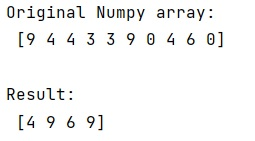# Get the n largest values of an array using NumPy

In this tutorial, we will learn how to get the n largest values of an array using NumPy? By Pranit Sharma Last updated : May 23, 2023

## Problem Statement

Given a NumPy array arr, and we have to get the n largest values from it. Where, n is 4.

## Solution to get the n largest values of an array using NumPy

To get the n largest values of an array using NumPy, you can simply use we will use np.argpartition(arr, -n)[-n:].

Let us understand with the help of an example,

## Example to get the n largest values of an array using NumPy

```# Import numpy
import numpy as np

# Creating a Numpy array
arr = np.array([9, 4, 4, 3, 3, 9, 0, 4, 6, 0])

# Display original numpy array
print("Original Numpy array:\n",arr,"\n")

# Defining a values for n
n = 4

# Using argpartition method for finding indices
# of n largest values
indx = np.argpartition(arr, -n)[-n:]

# Return indices
res = arr[indx]

# Display result
print("Result:\n",res)
```

### Output